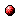# The QR decomposition and the singular value decomposition in the symmetrized max-plus algebra revisited

Reference:
B. De Schutter and B. De Moor, "The QR decomposition and the singular value decomposition in the symmetrized max-plus algebra revisited," SIAM Review, vol. 44, no. 3, pp. 417-454, 2002.

Abstract:
This paper is an updated and extended version of the paper "The QR decomposition and the singular value decomposition in the symmetrized max-plus algebra" (by B. De Schutter and B. De Moor, SIAM Journal on Matrix Analysis and Applications, vol. 19, no. 2, pp. 378-406, April 1998). The max-plus algebra, which has maximization and addition as its basic operations, can be used to describe and analyze certain classes of discrete-event systems, such as flexible manufacturing systems, railway networks, and parallel processor systems. In contrast to conventional algebra and conventional (linear) system theory, the max-plus algebra and the max-plus-algebraic system theory for discrete-event systems are at present far from fully developed and many fundamental problems still have to be solved. Currently, much research is going on to deal with these problems and to further extend the max-plus algebra and to develop a complete max-plus-algebraic system theory for discrete-event systems. In this paper we address one of the remaining gaps in the max-plus algebra by considering matrix decompositions in the symmetrized max-plus algebra. The symmetrized max-plus algebra is an extension of the max-plus algebra obtained by introducing a max-plus-algebraic analogue of the -operator. We show that we can use well-known linear algebra algorithms to prove the existence of max-plus-algebraic analogues of basic matrix decomposition from linear algebra such as the QR decomposition, the singular value decomposition, the Hessenberg decomposition, the LU decomposition, and so on. These max-plus-algebraic matrix decompositions could play an important role in the max-plus-algebraic system theory for discrete-event systems.

Downloads:Online version of the paperCorresponding technical report: pdf file (422 KB)
Note: More information on the pdf file format mentioned above can be found here.

Bibtex entry:

@article{DeSDeM:02-012,
author={B. {D}e Schutter and B. {D}e Moor},
title={The {QR} decomposition and the singular value decomposition in the symmetrized max-plus algebra revisited},
journal={SIAM Review},
volume={44},
number={3},
pages={417--454},
year={2002},
doi={10.1137/S00361445024039}
}

Go to the publications overview page.

This page is maintained by Bart De Schutter. Last update: August 12, 2020.